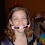## Wednesday, November 01, 2006

### Function of infinity

In the function f(x), f(x) equals the sum of the digits of x and the sum of the new number's digits and so on until one digit is remaining. For example:

f(787)=f(7+8+7)=f(22)=f(2+2)=f(4)=4
and
f(9135899)=f(9+1+3+5+8+9+9)= f(44)=f(4+4)=f(8)=8

So, if we assume that infinity is equal to 9999999999999... with an infinite amount of digits, then what is f(infinity)?

1.9

since the digits of every multiple of 9 always add to another multiple of 9.

2.Yes, Abe that would be the theoretical limit of the sum of infinity as defined as 999999...

But because infinity cannot effectively be quantified, and infinate number of 9's would still be infinity, there is no rational answer other than infinity.

3.I'd say you both got this one down.
f(infinity)=9

Any number with all nines for digits will all add up to nine:

f(9)=9
f(99)=f(18)=f(9)=9
f(999)=f(27)=f(9)=9
f(99999999999999)=f(126)=f(9)=9

Leave your answer or, if you want to post a question of your own, send me an e-mail. Look in the about section to find my e-mail address. If it's new, I'll post it soon.

Please don't leave spam or 'Awesome blog, come visit mine' messages. I'll delete them soon after.

Enter your Email and join hundreds of others who get their Question of the Day sent right to their mailbox

The Lamplight Manor Puzz 3-D